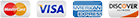# Convert XLSX to DOC Command Line

Total Excel Converter X is based on the award-winning functionality of our Total Excel Converter software and takes tabular data conversion to the next level by including support for web and server-based conversion.

You are free to integrate its power into your own applications due to its support for ActiveX or simply call it directly via the command line. As a command line XLSX converter, Total Excel Converter X puts an enormous range of functionality at your fingertips. If, for example, you are looking to convert XLSX to DOC via command line, it is a matter of just a few simple commands to convert one file or hundreds.

The full range of functionality that this powerful server XLSX converter puts at your disposal is sure to save you and your team an enormous amount of time. Here are just some of the advantages in using it:

• Support for all versions of Excel as well as OpenOffice and other input formats such as SQL and XML. New formats are constantly being added.
• Ability to combine several spreadsheets into a single file.
• Ability to rename files and edit header and footer settings. Dates, filenames and page counters can be added to each sheet.
• Take advantage of PDF security settings when converting to that format.
• Generate executable BAT files directly from the GUI if necessary.

One of the huge advantages of Total Excel Converter X is that your commands will run smoothly and without interruption so you will not waste time baby-sitting your conversion job runs or getting distracted by constant status update messages. Total Excel Converter X logs any error messages to an easily readable file so it is simple to troubleshoot individual conversion runs if anything goes wrong.

Total Excel Converter X is the perfect tool to take your document conversion workflow to the next level and step away from tedious manual processing for good. By leveraging the full power of this tool programmatically you will be saving yourself and your team an enormous amount of time and effort.

We encourage you to put the performance of Total Excel Converter X to the test before you purchase by checking out a fully functional, 30-day free trial today. Download your demo and start taking control of your tabular data today!## Examples of Total Excel ConverterX

### Convert excel files With TotalExcelConverterX and .NET

```string src="C:\\test\\Source.xlsx";
string dest="C:\\test\\Dest.PDF";

ExcelConverterX Cnv = new ExcelConverterX();
Cnv.Convert(src, dest, "-c PDF -log c:\\test\\Excel.log");

MessageBox.Show("Convert complete!");
```

### Convert Excel Files On Web Servers With Total Excel ConverterX

```dim C
Set C=CreateObject("ExcelConverter.ExcelConverterX")
C.Convert "c:\test\source.xlsx", "c:\test\dest.pdf", "-cPDF -log c:\test\Excel.log"
Response.Write C.ErrorMessage
set C = nothing
```
Example2 ASP: directly stream the resulting PDF
```dim C
Set C=CreateObject("ExcelConverter.ExcelConverterX")
Response.Clear
Response.BinaryWrite c.ConvertToStream("C:\www\ASP\Source.xlsx", "C:\www\ASP", "-cpdf  -log c:\html.log")
set C = nothing
```

### Convert Excel Spreadsheets by PHP With Total Excel ConverterX

```\$src="C:\\test\\test.XLS";
\$dest="C:\\test\\test.CSV";
\$c= new COM("ExcelConverter.ExcelConverterX");
\$c->convert(\$src,\$dest, "-c csv  -log c:\\test\\xls.log");
if (file_exists(\$dest)) echo "OK"; else echo "fail:".\$c->ErrorMessage;
```

### Convert Excel Spreadsheets By Ruby With Total Excel ConverterX

```require 'win32ole'
c = WIN32OLE.new('ExcelConverter.ExcelConverterX')

src="C:\\test\\test.xlsx";
dest="C:\\test\\test.pdf";

c.convert(src,dest, "-c PDF -log c:\\test\\Excel.log");

if not File.exist?(dest)
puts c.ErrorMessage
end

```

### Convert Excel Spreadsheets by Python With Total Excel ConverterX

```import win32com.client
import os.path

c = win32com.client.Dispatch("ExcelConverter.ExcelConverterX")

src="C:\\test\\test.xlsx";
dest="C:\\test\\test.pdf";

c.convert(src, dest, "-c PDF -log c:\\test\\Excel.log");

if not os.path.exists(file_path):
print(c.ErrorMessage)
```

### Convert Excel files With Pascal and Total Excel ConverterX

```uses Dialogs, Vcl.OleAuto;

var
c: OleVariant;
begin
c:=CreateOleObject('ExcelConverter.ExcelConverterX');
C.Convert('c:\test\source.xlsx', 'c:\test\dest.pdf', '-cPDF -log c:\test\Excel.log');
IF c.ErrorMessage<> Then
ShowMessage(c.ErrorMessage);
end;
```

### Convert Excel and ODS Files On Web Servers With Total Excel ConverterX

```var c = new ActiveXObject("ExcelConverter.ExcelConverterX");
c.Convert("C:\\test\\source.xlsx", "C:\\test\\dest.pdf", "-c PDF");
if (c.ErrorMessage!="")
```

### Convert Excel Spreadsheets by Perl With Total Excel ConverterX

```use Win32::OLE;

my \$src="C:\\test\\test.XLS";
my \$dest="C:\\test\\test.CSV";

my \$c = CreateObject Win32::OLE 'ExcelConverter.ExcelConverterX';
\$c->convert(\$src,\$dest, "-c csv  -log c:\\test\\xls.log");
print \$c->ErrorMessage if -e \$dest;
```Get all CoolUtils products
for \$99 only

(you save up to \$500)

### Latest News

• New Total PDF Printer has been released. Now you can print a folder of PDF files and then move the files to a different folder (-msuccess).
• We have fixed an issue in Coolutils Converter where some messages in HTML were not showing correctly.
• New Total HTML Converter has got an update with a few important bug fixes (large radius, paged layout).
• New Total Mail Converter Pro offers enhanced option to fit extra wide tables from emails to PDF files.
• New PDF Combine 7.0 is live. New options for creating combined PDF scans, e-books and songbooks.
• New Total Excel Converter 6.0 is live. Convert XLS with with charts.